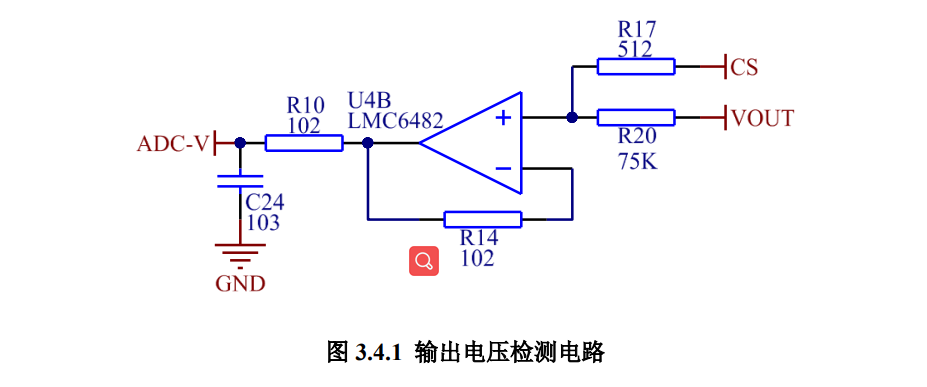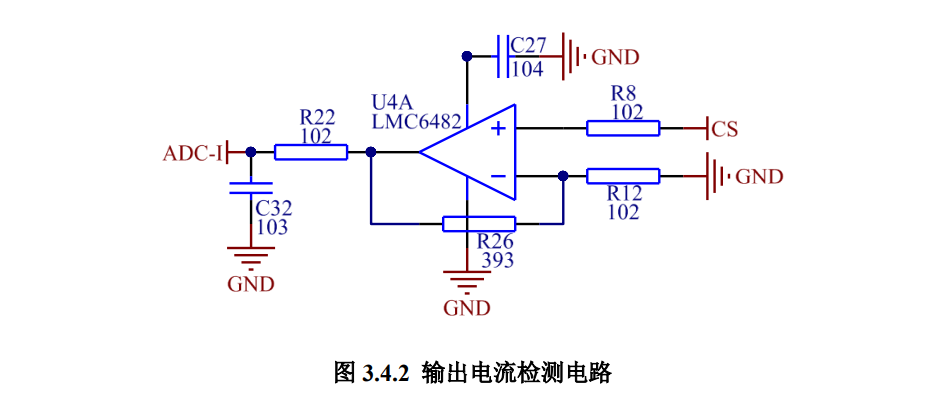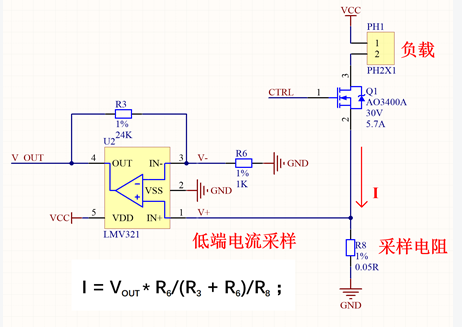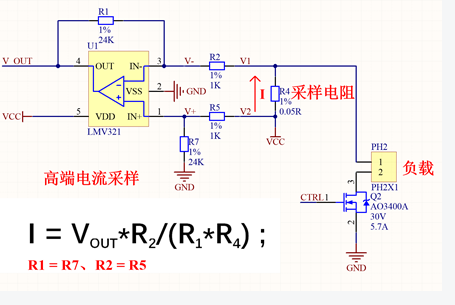# 输入输出电压检测# 输出电流检测➢1、低端运放电流检测方法：V+ = V-；

(VOUT-V-)/R3 = V-/R6；

VOUT = V+ * (R3 + R6)/R6；

V+ = I * R8

I =V+ / R8 = VOUT * R6/(R3 + R6)/R8

2、高端电流检测电路V+/R7 = （V2-V+)/R5；

V+=V-；

（V--VOUT)/R1 = （V1-V-)/R2；

VOUT = (V2-V1)*R1/R2；

V2-V1 = I*R4;

I = VOUT*R2/(R1*R4) ;

---------------------

THE END